Courses

# Thermodynamics MCQ - 2 (Advanced)

## 25 Questions MCQ Test Chemistry for JEE Advanced | Thermodynamics MCQ - 2 (Advanced)

Description
This mock test of Thermodynamics MCQ - 2 (Advanced) for JEE helps you for every JEE entrance exam. This contains 25 Multiple Choice Questions for JEE Thermodynamics MCQ - 2 (Advanced) (mcq) to study with solutions a complete question bank. The solved questions answers in this Thermodynamics MCQ - 2 (Advanced) quiz give you a good mix of easy questions and tough questions. JEE students definitely take this Thermodynamics MCQ - 2 (Advanced) exercise for a better result in the exam. You can find other Thermodynamics MCQ - 2 (Advanced) extra questions, long questions & short questions for JEE on EduRev as well by searching above.
*Multiple options can be correct
QUESTION: 1

### An ideal gas is taken from state A (Pressure P, Volume V) to the state B (Pressure P/2, Volume 2V) along a straight line path in PV diagram as shown in the adjacent figure.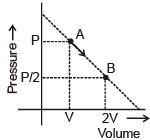Select the correct statement (s) among the following

Solution: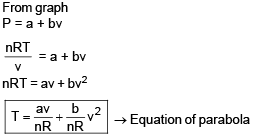*Multiple options can be correct
QUESTION: 2

### The normal boiling point of a liquid `A' is 350 K. ΔHvap at normal boiling point is 35 kJ/mole. Pick out the correct statement (s). (Assume ΔHvap to be independent of pressure).

Solution:
*Multiple options can be correct
QUESTION: 3

### Which of the following statement(s) is/are false :

Solution:

For reversible process
(ΔS)sys = 0 (at const T & P)
(ΔS)T is maximised at equation
ΔGº = f(T) (For an ideal gas)

*Multiple options can be correct
QUESTION: 4

Which statement is/are correct :

Solution:
*Multiple options can be correct
QUESTION: 5

Which is /are correct statement.

Solution:

At high temperature vibrational degree of freedom is active.

*Multiple options can be correct
QUESTION: 6

Two moles of an ideal gas (Cv,m = 3/2 R) is subjected to following change of state.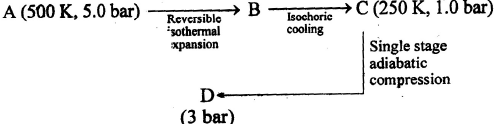The correct statement is/are :

Solution: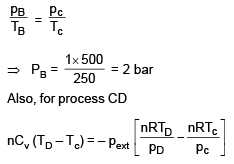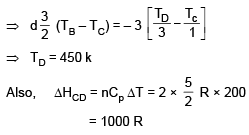*Multiple options can be correct
QUESTION: 7

In isothermal ideal gas compression

Solution:

ΔG = – TΔS (ΔS, ΔH = 0)
As ΔS < 0
ΔG > 0

*Multiple options can be correct
QUESTION: 8

A piston cyclinder device initially contains 0.2 m3 neon (assume ideal) at 200 kPa inside at T1°C. A valve is now opened and neon is allowed to escape until the volume reduces to half the initial volume. At the same time heat transfer with outside at T2° C ensures a constant temperature inside.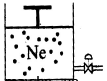Select correct statement(s) for given process

Solution:

we know that internal energy is an extensive property so we can't comment on ΔU

*Multiple options can be correct
QUESTION: 9

If one mole monoatomic ideal gas was taken through process AB as shown in figure, the select correct option (s).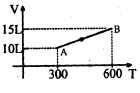Solution: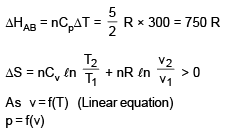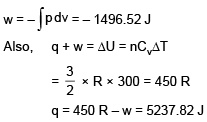*Multiple options can be correct
QUESTION: 10

Which of the following statement(s) is/are correct

Solution: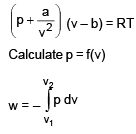QUESTION: 11

A cylindrical container of volume 44.8 litres is containing equal no. of moles (in integer no.) of an ideal monoatomic gas in two sections A and B separated by an adiabatic frictionless piston as shown in figure. The initial temperature and pressure of gas in both section is 27.3 K and 1 atm. Now gas is section `A' is slowly heated till the volume of section B becomes (1/8)th of initial volume.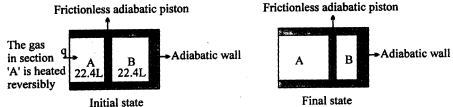Q.

What will be the final pressure in container B.

Solution:
QUESTION: 12

A cylindrical container of volume 44.8 litres is containing equal no. of moles (in integer no.) of an ideal monoatomic gas in two sections A and B separated by an adiabatic frictionless piston as shown in figure. The initial temperature and pressure of gas in both section is 27.3 K and 1 atm. Now gas is section `A' is slowly heated till the volume of section B becomes (1/8)th of initial volume.Q.

Final temperature in container A will be

Solution:
QUESTION: 13

A cylindrical container of volume 44.8 litres is containing equal no. of moles (in integer no.) of an ideal monoatomic gas in two sections A and B separated by an adiabatic frictionless piston as shown in figure. The initial temperature and pressure of gas in both section is 27.3 K and 1 atm. Now gas is section `A' is slowly heated till the volume of section B becomes (1/8)th of initial volume.Q.

Change in enthalpy for section A in Kcal.

Solution:
QUESTION: 14

The vapour pressure of H2O(l) at 353 K is 532 mm Hg. The external pressure on H2O (l) taken in a cylinder fitted with frictionless movable piston initially containing 0.9 L (= 0.9 kg) of H2O (l) at 353 K is increased to 1 atm. Temperature remained constant. Now, heat is supplied keeping pressure constant till 0.45 L of H2O (l) = (0.45 kg) is evaporated to form H2O (g) at 373 K. Carefully observe the diagrams provided and from given data, answer the following questions.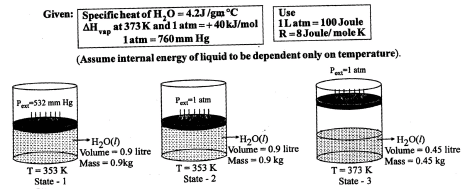Q.

ΔH when system is taken from state 1 to state 2 (Joule) ?

Solution:
QUESTION: 15

The vapour pressure of H2O(l) at 353 K is 532 mm Hg. The external pressure on H2O (l) taken in a cylinder fitted with frictionless movable piston initially containing 0.9 L (= 0.9 kg) of H2O (l) at 353 K is increased to 1 atm. Temperature remained constant. Now, heat is supplied keeping pressure constant till 0.45 L of H2O (l) = (0.45 kg) is evaporated to form H2O (g) at 373 K. Carefully observe the diagrams provided and from given data, answer the following questions.Q.

Total change in DU going from state 1 to 3 (kJ) ?

Solution:
QUESTION: 16

The vapour pressure of H2O(l) at 353 K is 532 mm Hg. The external pressure on H2O (l) taken in a cylinder fitted with frictionless movable piston initially containing 0.9 L (= 0.9 kg) of H2O (l) at 353 K is increased to 1 atm. Temperature remained constant. Now, heat is supplied keeping pressure constant till 0.45 L of H2O (l) = (0.45 kg) is evaporated to form H2O (g) at 373 K. Carefully observe the diagrams provided and from given data, answer the following questions.Q.

Total change in enthalpy going from state 1 to state 3 (kJ) ?

Solution:
QUESTION: 17

The vapour pressure of H2O(l) at 353 K is 532 mm Hg. The external pressure on H2O (l) taken in a cylinder fitted with frictionless movable piston initially containing 0.9 L (= 0.9 kg) of H2O (l) at 353 K is increased to 1 atm. Temperature remained constant. Now, heat is supplied keeping pressure constant till 0.45 L of H2O (l) = (0.45 kg) is evaporated to form H2O (g) at 373 K. Carefully observe the diagrams provided and from given data, answer the following questions.Q.

What is the work done in going state 1 to state 3 to in Joules.

Solution:
QUESTION: 18

comprehension-3

Two moles of helium gas are taken over the cycle ABCDA, as shown in the P-T diagram.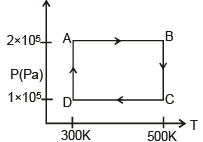Q.

Assuming the gas to be ideal the work done by the gas in taking it from A to B is -

Solution:
QUESTION: 19

Two moles of helium gas are taken over the cycle ABCDA, as shown in the P-T diagram.Q.

The work done involved in taking it from D to A is

Solution:
QUESTION: 20

Two moles of helium gas are taken over the cycle ABCDA, as shown in the P-T diagram.Q.

The net work done involved in the cycle ABCDA is

Solution:
QUESTION: 21

Match Column-I with Column-II

Column - I Column-II

(Ideal Gas) (Related equation)

(A) Reversible isothermal (P) W = 2.303 nRT log (P2/P1) process

(B) Reversible adiabatic (Q) W = nCV,m (T2 _ T1) process

(C) Irreversible adiabatic (R) PV = nRT process

(D) Irreversible isothermal (S) process

Solution:
QUESTION: 22

Match the column - I with column-II

Note that column - I may have more than one matching options in column-II

Column-I Column-II

(A) Reversible adiabatic compression (P) DSsystem > 0

(B) Reversible vaporisation (Q) DSsystem < 0

(C) Free expansion of ideal gas in vacuum (R) DSsurroudning < 0

(D) Dissociation of CaCO3(s) → CaO(s) + CO2(g) (S) DSsurrounding = 0

Solution:
QUESTION: 23

Statement-1 : There is no change in enthalpy of an ideal gas during compression at constant temperautre.

Statement-II : Enthalpy of an ideal gas is a function of temperature and pressure.

Solution:
QUESTION: 24

Statement-1 : Due to adiabatic free expansion, temperature of a real gas always increases

Statement-II : If a real gas is at inversion temperature then no change in temperature is observed in adiabatic free expansion.

Solution:
QUESTION: 25

Statement-1 : There is no change in enthalpy of an ideal gas during compression at constant pressure.

Statement-II : Enthalpy of an ideal gas is a function of temperature .

Solution: